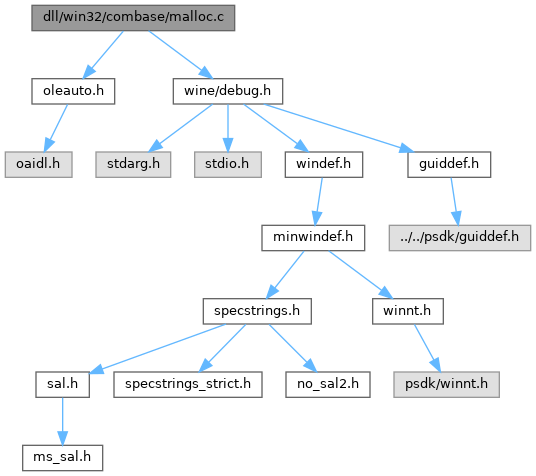ReactOS 0.4.15-dev-6679-g945ee4b
malloc.c File Reference
`#include <precomp.h>`
`#include <stdlib.h>`
`#include <malloc.h>`
Include dependency graph for malloc.c:Go to the source code of this file.

## Macros

#define ROUND_DOWN(n, align)    (((ULONG)n) & ~((align) - 1l))

#define ROUND_UP(n, align)    ROUND_DOWN(((ULONG)n) + (align) - 1, (align))

#define ROUND_SIZE(size)   (max(16, ROUND_UP(size, 16)))

## Functions

voidmalloc (size_t _size)

void free (void *_ptr)

voidcalloc (size_t _nmemb, size_t _size)

voidrealloc (void *_ptr, size_t _size)

void_expand (void *_ptr, size_t _size)

size_t _msize (void *_ptr)

int _heapchk (void)

int _heapmin (void)

int _heapset (unsigned int unFill)

int _heapwalk (struct _heapinfo *entry)

## ◆ ROUND_DOWN

 #define ROUND_DOWN ( n, align ) (((ULONG)n) & ~((align) - 1l))

Definition at line 28 of file malloc.c.

## ◆ ROUND_SIZE

 #define ROUND_SIZE ( size ) (max(16, ROUND_UP(size, 16)))

Definition at line 35 of file malloc.c.

## ◆ ROUND_UP

 #define ROUND_UP ( n, align ) ROUND_DOWN(((ULONG)n) + (align) - 1, (align))

Definition at line 31 of file malloc.c.

## ◆ _expand()

 void * _expand ( void * _ptr, size_t _size )

Definition at line 100 of file malloc.c.

101{
102 size_t nSize;
103
104 nSize = ROUND_SIZE(_size);
105
106 if (nSize<_size)
107 return NULL;
108
110}
#define NULL
Definition: types.h:112
#define GetProcessHeap()
Definition: compat.h:736
#define HeapReAlloc
Definition: compat.h:734
#define ROUND_SIZE(size)
Definition: malloc.c:35
#define HEAP_REALLOC_IN_PLACE_ONLY
Definition: nt_native.h:1696
*nSize LPSTR _Inout_ LPDWORD nSize
Definition: winbase.h:2074

## ◆ _heapchk()

 int _heapchk ( void )

Definition at line 123 of file malloc.c.

124{
125 if (!HeapValidate(GetProcessHeap(), 0, NULL))
126 return -1;
127 return 0;
128}
BOOL WINAPI HeapValidate(HANDLE hHeap, DWORD dwFlags, LPCVOID lpMem)
Definition: heapmem.c:156

Referenced by _heapset().

## ◆ _heapmin()

 int _heapmin ( void )

Definition at line 133 of file malloc.c.

134{
135 if (!HeapCompact(GetProcessHeap(), 0))
136 return -1;
137 return 0;
138}
SIZE_T WINAPI HeapCompact(HANDLE hHeap, DWORD dwFlags)
Definition: heapmem.c:145

## ◆ _heapset()

 int _heapset ( unsigned int unFill )

Definition at line 143 of file malloc.c.

144{
145 if (_heapchk() == -1)
146 return -1;
147 return 0;
148
149}
int _heapchk(void)
Definition: malloc.c:123

## ◆ _heapwalk()

 int _heapwalk ( struct _heapinfo * entry )

Definition at line 154 of file malloc.c.

155{
156 return 0;
157}

## ◆ _msize()

 size_t _msize ( void * _ptr )

Definition at line 115 of file malloc.c.

116{
117 return HeapSize(GetProcessHeap(), 0, _ptr);
118}
SIZE_T WINAPI HeapSize(HANDLE, DWORD, LPCVOID)

## ◆ calloc()

 void * calloc ( size_t _nmemb, size_t _size )

Definition at line 61 of file malloc.c.

62{
63 size_t nSize = _nmemb * _size;
64 size_t cSize = ROUND_SIZE(nSize);
65
66 if ( (_nmemb > ((size_t)-1 / _size)) || (cSize<nSize))
67 return NULL;
68
69 return HeapAlloc(GetProcessHeap(), HEAP_ZERO_MEMORY, cSize );
70}
#define HeapAlloc
Definition: compat.h:733
#define HEAP_ZERO_MEMORY
Definition: compat.h:134

## ◆ free()

 void free ( void * _ptr )

Definition at line 53 of file malloc.c.

54{
55 HeapFree(GetProcessHeap(),0,_ptr);
56}
#define HeapFree(x, y, z)
Definition: compat.h:735

## ◆ malloc()

 void * malloc ( size_t _size )

Definition at line 40 of file malloc.c.

41{
42 size_t nSize = ROUND_SIZE(_size);
43
44 if (nSize<_size)
45 return NULL;
46
47 return HeapAlloc(GetProcessHeap(), 0, nSize);
48}

## ◆ realloc()

 void * realloc ( void * _ptr, size_t _size )

Definition at line 75 of file malloc.c.

76{
77 size_t nSize;
78
79 if (_ptr == NULL)
80 return malloc(_size);
81
82 if (_size == 0)
83 {
84 free(_ptr);
85 return NULL;
86 }
87
88 nSize = ROUND_SIZE(_size);
89
90 /* check for integer overflow */
91 if (nSize<_size)
92 return NULL;
93
94 return HeapReAlloc(GetProcessHeap(), 0, _ptr, nSize);
95}
#define free
Definition: debug_ros.c:5
#define malloc
Definition: debug_ros.c:4